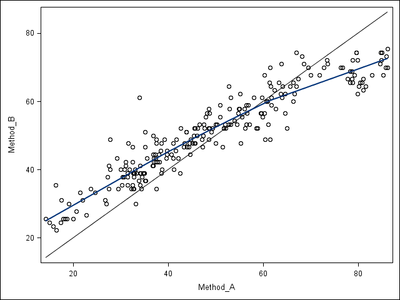## Data Transformation_normality

Hi All,

The attached file contains moisture content in percent measured using two methods. Data of both the methods is not normal. I need to develop a Bland and Altman graph on the difference (diffs) of both the methods that require the diffs to be normally distributed. I tried various log, log10, sqrt, and square to transform the original data but they don't improve the normality. The Shapiro-Wilks test rejects the normality, but visually data seems to be fine except for the diffs.

Kindly suggest me how to improve normality of the data with the SAS codes if possible. Thank you very much

3 REPLIES 3PGStats
Opal | Level 21

## Re: Data Transformation_normality

Normality is not the problem here. There is a non trivial relationship between the two measurement methods that you should not ignore.What is the goal of your analysis?

Note: The graph was made with:

``````proc sort data=trans; by method_a;

proc sgplot data=trans noautolegend;
scatter x=method_a y=method_b;
series x=method_a y=method_a;
loess x=method_a y=method_b / smooth=0.7;
run;
``````
PG

## Re: Data Transformation_normality

Hi PG,

Thank you very much for your input on this. Sorry for a delayed response, I opened this message previously on my cell phone where the graph was not visible. But, now on my computer, I could see, and it caught my attention.

Let me pick your brain; what is that non-trivial relationship between the two measurement methods, please?

(Measurement with Method_A is based on calibration and Method_B is a gold standard method measurement. Moreover (This is what I see): method_A underestimate at higher concentration while over-estimate at low concentration!!!?).

Before, I answer your question about my goal for the analysis. A little background on the dataset:.  Apart from the dataset that you see, I have another independent data on two paired measurements (TEMR and TEM%) on a  population (N-238) to develop a regression model. There is a published regression model (TEM%=1.11*TEMR), and  I have used the published regression coefficient (y=1.11) on my TEMR  readings to get  "Method_A" and have compared as shown in the graph with my TEM% readings "Method_B."

My goal of the analysis is to demonstrate graphically that measurement by the methods differ (I have developed Bland and Altman graphs) and I don't know yet how to compare the methods statistically, probably will do a paired t-test (any thought on this, please?)

I am also thinking of comparing the slope of the published regression model with the regression model that I am developing. Please, advise how I can do a "Chow test" (requesting SAS code) on the two regression models to see if the slopes differ? (I don't have access to the published data; just the regression coefficient).

Lastly, how have you developed the trend line (SAS code of the gplot, please) on the data point in the graph?

Thank you very much again

Fridge_wpgKsharp
Super User

## Re: Data Transformation_normality

```Check PROC MCMC

```
Discussion stats
• 3 replies
• 738 views
• 1 like
• 3 in conversation